# ISEE Lower Level Math : Geometry

## Example Questions

### Example Question #48 : How To Find The Area Of A Rectangle

Find the area of a rectangle with a length of 10 inches and a width that is half the length.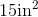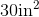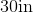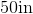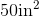Explanation:

To find the area of a rectangle, we will use the following formula: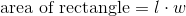where l is the length and w is the width of the rectangle.

Now, we know the length of the rectangle is 10 inches.  We also know the width is half the length.  Therefore, the width is 5 inches.  Knowing this, we can substitute into the formula.  We get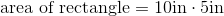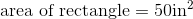### Example Question #49 : How To Find The Area Of A Rectangle

Find the area of a rectangle with a length of 8in and a width of 7in.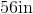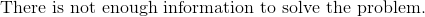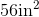Explanation:

To find the area of a rectangle, we will use the following formula:where l is the length and w is the width of the rectangle.

Now, we know the length is 8in and the width is 7in.  Knowing this, we can substitute into the formula.  We get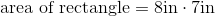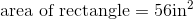### Example Question #51 : Rectangles

Find the area of a rectangle with a length of 7cm and a width of 3cm.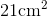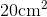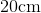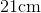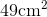Explanation:

To find the area of a rectangle, we will use the following formula:where l is the length and w is the width of the rectangle.

Now, we know the length of the rectangle is 7cm.  We also know the width of the triangle is 3cm.  Knowing this, we can substitute into the formula.  We get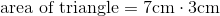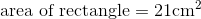### Example Question #51 : How To Find The Area Of A Rectangle

Use the following figure to answer the question: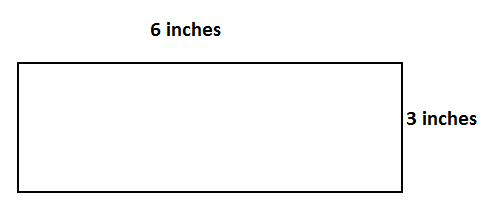Find the area.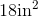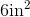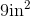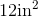Explanation:

To find the area of a rectangle, we will use the following formula:where l and w are the length and the width of the rectangle.

Now, let's look at the rectangleWe can see the length, or the long side, is 6 inches.  We can also see the width, or the short side, is 3 inches.

Knowing this, we can substitute into the formula.  We get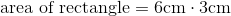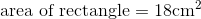### Example Question #52 : How To Find The Area Of A Rectangle

Find the area of a rectangle with a length of 8 feet and a width of 7 feet.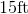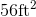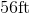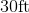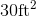Explanation:

To find the area of a rectangle, we will use the following formula:where l is the length and w is the width of the rectangle.

Now, we know the length of the rectangle is 8ft.  We also know the width of the rectangle is 7ft.

Knowing this, we will substitute into the formula.  We get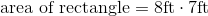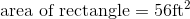### Example Question #54 : How To Find The Area Of A Rectangle

Use the following rectangle to answer the question: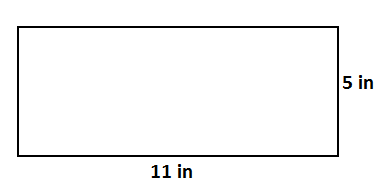Find the area.Explanation:

To find the area of a rectangle, we will use the following formula:where l is the length and w is the width of the rectangle.

Now, given the rectanglewe can see the length is 11in and the width is 5in.

Knowing this, we can substitute into the formula.  We get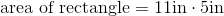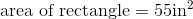### Example Question #55 : How To Find The Area Of A Rectangle

Find the area of a rectangle with a length of 8cm and a width of 6cm.Explanation:

To find the area of a rectangle, we will use the following formula:where l is the length and w is the width of the rectangle.

Now, we know the length of the rectangle is 8cm.  We also know the width of the rectangle is 6cm.  Knowing this, we can substitute into the formula.  We get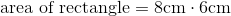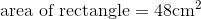### Example Question #56 : How To Find The Area Of A Rectangle

Use the following rectangle to answer the question:Find the area.Explanation:

To find the area of a rectangle, we will use the following formula:where l is the length and w is the width of the rectangle.

Now, let's look at the rectangle.We can see the length is 6 inches.  We can also see the width is 3 inches.

Knowing this, we can substitute into the formula.  We get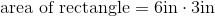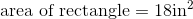### Example Question #53 : How To Find The Area Of A Rectangle

Find the area of a rectangle with a width of 5cm and a length that is two times the width.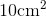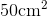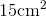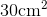Explanation:

To find the area of a rectangle, we will use the following formula:where is the length and w is the width of the rectangle.

Now, we know the width of the rectangle is 5cm.  We also know the length is two times the width.  Therefore, the length is 10cm.

Knowing this, we can substitute into the formula.  We get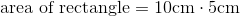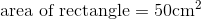### Example Question #1021 : Isee Lower Level (Grades 5 6) Mathematics Achievement

Use the following image to answer the question: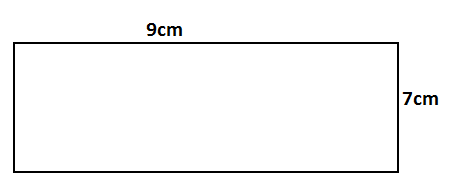Find the area.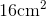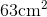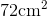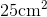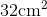Explanation:

To find the area of a rectangle, we will use the following formula:where l is the length and w is the width of the rectangle.

Now, given the rectangleWe can see the length is 9cm.  We can also see the width is 7cm.

Knowing this, we can substitute into the formula.  We get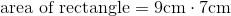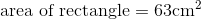### All ISEE Lower Level Math Resources